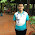## Thursday, October 20, 2011

### No. 08 - Calculate 1+2+…+n

Problem: Calculate 1+2+…+n without multiplication, division, key words for, while, if, else, switch, case, as well as conditional operator (A ? B : C).

Analysis: This problem is not meaningful during software development since usually we do not have such rigorous limitations. However, many interviewers believe that it is useful to test candidates’ ability of divergent thinking. Ability of divergent thinking reflects the depth and width of programming understanding.

Besides equation n(n+1)/2 to get 1+2+…+n, we only have two approaches: Iteration and recursion. Since key words for and while are forbidden, we cannot utilize iteration directly any more. In a recursive function, we need to use key word if or conditional operators to check whether we should continue or stop recursion. Unfortunately, both of them are also forbidden.

Solution 1: Based on Constructors

Let us firstly focus on iterations. An iteration is actually only to repeat n times, and we can achieve it without key words for and while. We can define a class, and then create n instances of it. Therefore, its constructor and destructor will be definitely called n times. If we implement calculation operations inside the constructor, it will iterate for n times. The following code is based on this solution:

class Temp
{
public:
Temp() { ++ N; Sum += N; }

static void Reset() { N = 0; Sum = 0; }
static unsigned int GetSum() { return Sum; }

private:
static unsigned int N;
static unsigned int Sum;
};

unsigned int Temp::N = 0;
unsigned int Temp::Sum = 0;

unsigned int Sum_Solution1(unsigned int n)
{
Temp::Reset();

Temp *a = new Temp[n];
delete []a;
a = NULL;

return Temp::GetSum();
}

Solution 2: Based on Virtual Functions

We secondly focus on recursion. We cannot determine to continue or stop recursion inside a single function. How about to define two functions, one for normal operations and the other as a terminator? We may use Boolean variables since we are going to select a function out of two. When the Boolean variable is true (1), the operational function will be selected. When it is false (0), the terminal function will be selected.

We have to convert integer variables into Boolean variables. It is an easy task since it can be achieved with two not operations (!!n). Non-zero numbers will be true with two not operations and zero will be false.

class A;
A* Array;

class A
{
public:
virtual unsigned int Sum (unsigned int n)
{
return 0;
}
};

class B: public A
{
public:
virtual unsigned int Sum (unsigned int n)
{
return Array[!!n]->Sum(n-1) + n;
}
};

int Sum_Solution2(int n)
{
A a;
B b;
Array = &a;
Array = &b;

int value = Array->Sum(n);

return value;
}

This solution is based on virtual functions. The function B::Sum is called when variable n is not zero, while the function A::Sum, which acts as a terminator, is called when n equals to zero.

Solution 3: Based on Function Pointers

There are no virtual functions in native C programming environment, so we have to simulate them with function pointers. The code below may be more straightforward:
typedef unsigned int (*fun)(unsigned int);

unsigned int Solution3_Teminator(unsigned int n)
{
return 0;
}

unsigned int Sum_Solution3(unsigned int n)
{
static fun f = {Solution3_Teminator, Sum_Solution3};
return n + f[!!n](n - 1);
}

Solution 4: Based on Template Classes

We can also utilize compiler to simulate recursive calculate. Let us have a look at the following code:
template <unsigned int n> struct Sum_Solution4
{
enum Value { N = Sum_Solution4<n - 1>::N + n};
};

template <> struct Sum_Solution4<1>
{
enum Value { N = 1};
};

The value of Sum_Solution4<100>::N is the result of 1+2+…+100. When compilers see Sum_Solution4<100>, it will generate code for the template class Sum_Solution4 with parameter 100. A class Sum _Solution4 <99> is needed to generate the class Sum_Solution4<100> since Sum _Solution4<100>::N= Sum _Solution4 <99>::N+100. The recursive process stops when it reaches the Sum_Solution4<1> because it has been defined explicitly.

In the solution, the input n must be a constant value since calculations are all in compiling time. It is a big short coming for this solution. Additionally, n cannot be a large number since compilers have limitations on the depths of recursive compiling.

The discussion about this problem is included in my book <Coding Interviews: Questions, Analysis & Solutions>, with some revisions. You may find the details of this book on Amazon.com, or Apress.

The author Harry He owns all the rights of this post. If you are going to use part of or the whole of this ariticle in your blog or webpages,  please add a referenced to http://codercareer.blogspot.com/. If you are going to use it in your books, please contact me (zhedahht@gmail.com) . Thanks.

1.2.It's contains very useful data which i need and i want to see more quality posts in this blog so please update your blog. Thanks for sharing. c programming homework help

3.return (sqr(n)+n)>>1;

4.int sum( int n)
{
int l = 1;
(n == 1) || (l = sum(n-1) + n);
return l;
}

5.